# ISEE Middle Level Quantitative : How to find the answer from a table

## Example Questions

← Previous 1 3 4

### Example Question #1 : Tables

Choose the best answer from the four choices given.

Katie wants to buy as many umbrellas as she can to give to her friends as Christmas gifts. She finds a deal online where she can get umbrellas for $7 each or four umbrellas for$25 (including tax). If shipping is $3 for every$20 of merchandise (or portion thereof), how many umbrellas can she buy if she has $140? Possible Answers: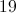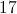Correct answer:Explanation: The quickest way to solve this problem is by making a chart showing the units purchased and the total cost. Start with the 4-packs of umbrellas: 4-packs cost shipping cost total cost 1$25               $6$31

2                $50$9                          $59 3$75               $12$87

4                $100$15                        $115 5$125             $21$146 (too high)

Having determined that Katie can buy four of the 4-packs of umbrellas, now we see how many of the $7 individual umbrellas she can add to her order. four 4-packs indiv. indiv.$     subtotal     shipping $total$

$100 1$7           $107$18          $125$100                      2               $14$114         $18$132

$100 3$21         $121$21          $142 (too high) Therefore, Katie can buy (4) 4-packs and (2) individual umbrellas (18 total umbrellas) for$132.

ALTERNATIVELY, you can begin by determining the highest subtotal she can afford and work backwards from there. $120 is 6 x$20, so the shipping charge would be $6 x 3 =$18, for a total cost of $138. One more dollar on the subtotal would bring it into the next$20 segment, bringing the shipping charge up to $21 (and$142 is greater than $140). Thus, from here you could figure out the maximum number of$25 4-packs and $7 individual umbrellas Katie could buy with$120.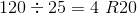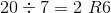Since \$6 is not enough to buy any umbrellas, the total would be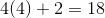### Example Question #2 : How To Find The Answer From A Table

The table below gives the population of Buchanan City in each census year from 1930  to 1980.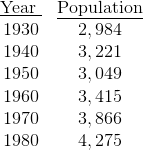Which is the greater quantity?

(a) The decline in the population of Buchanan City between 1940 and 1950

(b) The growth in the population of Buchanan City between 1950 and 1960

(a) is greater

It is impossible to tell from the information given

(a) and (b) are equal

(b) is greater

(b) is greater

Explanation:

The population of Buchanan City in 1960 is greater than it was in 1940, so its decline between 1940 and 1950 must be less than its growth between 1950 and 1960.

### Example Question #1 : How To Find The Answer From A Table

The table below gives the population of Buchanan City in each census year from 1930  to 1980.Which is the greater quantity?

(a) The growth in the population of Buchanan City between 1930 and 1940

(b) The growth in the population of Buchanan City between 1950 and 1960

(a) and (b) are equal

It is impossible to tell from the information given

(b) is greater

(a) is greater

(b) is greater

Explanation:

(a) Between 1930 and 1940, the population grew by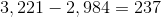(b) Between 1950 and 1960, the population grew by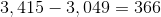.

This makes (b) greater

### Example Question #1 : Tables

The table below gives the population of Buchanan City in each census year from 1930  to 1980.The population figures are not available for 1990, 2000, or 2010, but it is known that the population has not decreased since 1980.

Which is the greater quantity?

(a) The population of Buchanan City in 2010

(b)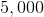(a) is greater

It is impossible to tell from the information given

(b) is greater

(a) and (b) are equal

It is impossible to tell from the information given

Explanation:

Since the population has not decreased since 1980, it can be inferred that the population in 2010 was greater than or equal to that in 1980, which is 4,275. However, nothing more is known.

### Example Question #5 : How To Find The Answer From A Table

The table below gives the population of Buchanan City in each census year from 1930  to 1980.In 1930, 81% of the population of Buchanan City was non-White; in 1980, 61% was non-White.

Which is the greater quantity?

(a) The number of non-Whites living in Buchanan City in 1930

(b) The number of non-Whites living in Buchanan City in 1980

It is impossible to tell from the information given

(a) is greater

(b) is greater

(a) and (b) are equal

(b) is greater

Explanation:

(a) 81% of 2,984 is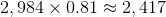(b) 61% of 4,275 is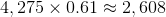(b) is greater

### Example Question #91 : Data Analysis

The table below gives the population of Buchanan City in each census year from 1930  to 1980.The population figures are not available for 1990, 2000, or 2010, but it is known that the population has increased by at least 300 over each ten-year period since 1980.

Which is the greater quantity?

(a) The population of Buchanan City in 2010

(b)(a) is greater

It is impossible to tell from the information given

(a) and (b) are equal

(b) is greater

(a) is greater

Explanation:

Since the population of Buchanan City increased by at least 300 over each of three decades since 1980, then the population in 2010 is at least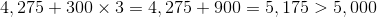.

### Example Question #1 : Tables

The table below gives the population of Buchanan City in each census year from 1930  to 1980.Which is the greater quantity?

(a) The population of Buchanan City in 1965

(b) The population of Buchanan City in 1975

(a) and (b) are equal

It is impossible to tell from the information given

(a) is greater

(b) is greater

It is impossible to tell from the information given

Explanation:

No information about the population of Buchanan City is given for either 1965 or 1975.

### Example Question #2 : Tables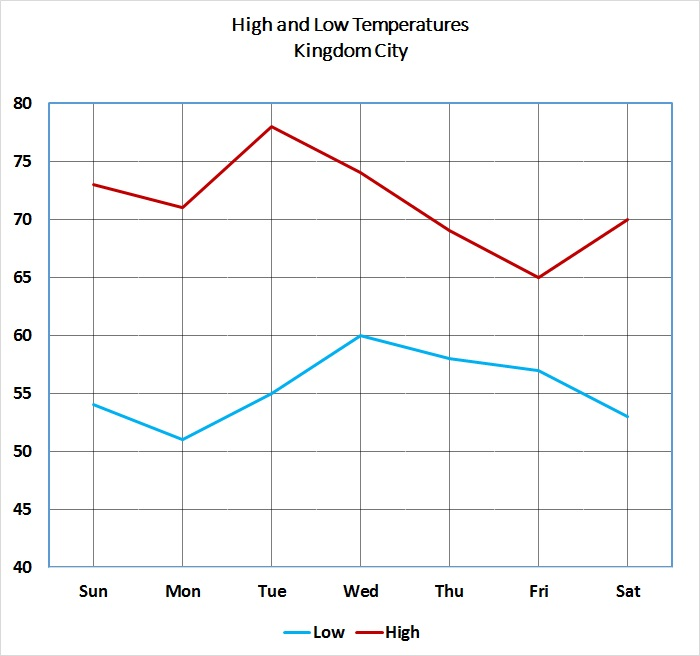Refer to the above graph, which shows the high and low temperatures for Kingdom City over a one-week period.

On how many days did the temperature fall below 55 degrees?

Three

One

Two

Four

Three

Explanation:

To answer this question, examine the line representing the low temperatures.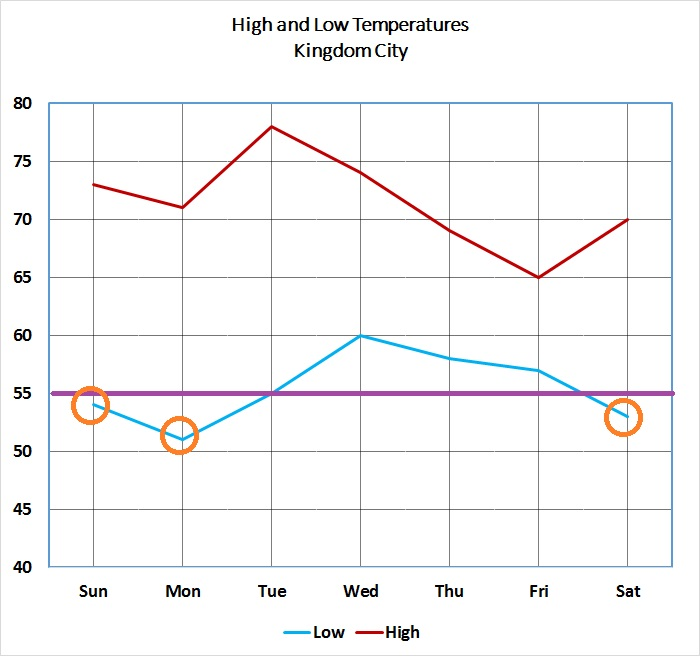Notice that the three days on which the temperature fell below 55 have been marked with a circle (the low temperature on Tuesday was exactly 55).

### Example Question #1 : TablesRefer to the above graph, which shows the high and low temperatures for Kingdom City over a one-week period.

On how many days did the difference between the high and low temperatures reach or exceed fifteen degrees?

Four

Seven

Six

Five

Four

Explanation:

Below is the graph, this time with the temperatures represented.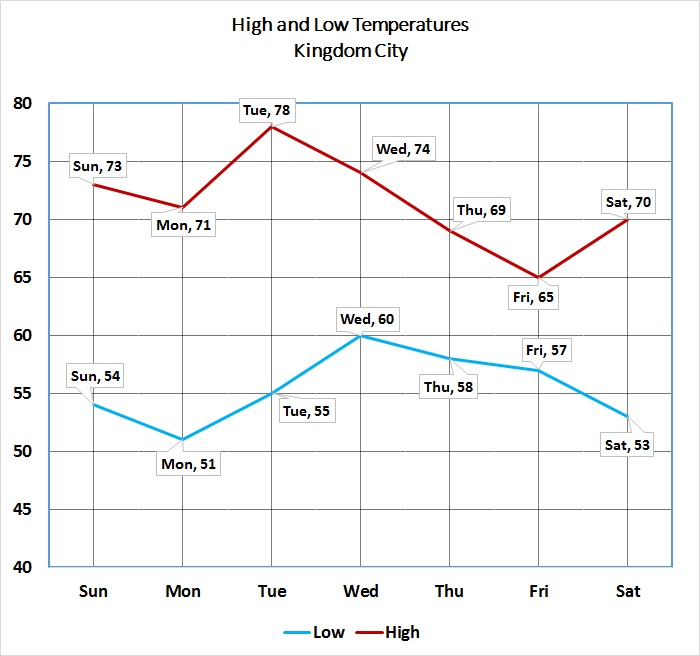The differences between the high and low temperatures for each day are as follows:

Sunday: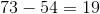Monday: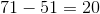Tuesday: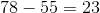Wednesday: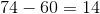Thursday: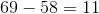Friday: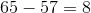Saturday: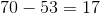The differences for four days - Sunday, Monday, Tuesday, and Saturday - all exceed 15 degrees.

### Example Question #3 : Tables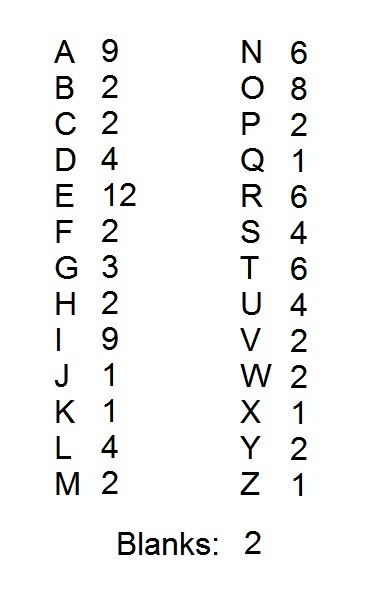A popular word game uses one hundred tiles, each of which is marked with a letter or a blank. The distribution of the tiles is shown above, with each letter paired with the number of tiles marked with that letter. Notice that there are two blank tiles.

How many consonants are represented by as many tiles as there are "U" tiles?

(Note: for this problem, "Y" is considered a consonant)

Two

Four

Five

Three